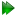Subsections

value.writeSynopsis

Write spin specific parameter values to a file.

Defaults

value.write(param=None, file=None, dir=None, scaling=1.0, comment=None, bc=False, force=False)

Keyword arguments

param: The parameter.

file: The name of the file.

dir: The directory name.

scaling: The factor to scale parameters by.

comment: Text which will be added to the start of the file as comments. All lines will be prefixed by `# '.

bc: A flag which if True will cause the back calculated values to be written to file rather than the actual data.

force: A flag which, if set to True, will cause the file to be overwritten.

Description

The values corresponding to the given parameter will be written to file. The scaling argument can be used to scale the parameter values. This can be useful for example in the case of the model-free Rex parameter to obtain the spectrometer dependent value from the omega_ex field strength independent internal value. Or to scale correlation times from seconds down to nanosecond or picosecond timescales.

Relaxation curve fitting parameters

Please see Table 17.33 on page.

Please see Table 17.9 on page.

Model-free parameters

Please see Table 17.34 on page.

For model-free theory it is assumed that Rex values are scaled quadratically with field strength. The values will be very small as they will be written out as a field strength independent value. Hence use the following formula to convert the value to that expected for a given magnetic field strength:

Rex = value * (2.0 * pi * frequency) ** 2

The frequency is that of the proton in Hertz.

Reduced spectral density mapping parameters

Please see Table 17.11 on page.

Consistency testing parameters

Please see Table 17.12 on page.

Relaxation dispersion parameters

Please see Table 17.5 on page.

Prompt examples

To write the CSA values to the file `csa.txt', type one of:

[numbers=none]
relax> value.write('csa', 'csa.txt')

[numbers=none]
relax> value.write(param='csa', file='csa.txt')

To write the NOE values to the file `noe', type one of:

[numbers=none]
relax> value.write('noe', 'noe.out')

[numbers=none]
relax> value.write(param='noe', file='noe.out')

[numbers=none]
relax> value.write(param='noe', file='noe.out')

[numbers=none]
relax> value.write(param='noe', file='noe.out', force=True)

To write the model-free Rex values scaled to 600 MHz to the file `rex_600', type one of:

[numbers=none]
relax> value.write('rex', 'rex_600', scaling=(2.0*pi*600e6)**2)

[numbers=none]
relax> value.write(param='rex', file='rex_600', scaling=(2.0*pi*600e6)**2)

The relax user manual (PDF), created 2020-08-26.